# Introduction to Linear Regression

Hi everyone,

Welcome to my Introduction to Linear Regression blog.

Linear regression is arguably the most popular Machine learning model out there. Among machine learning courses and textbooks, Linear regression is often (or maybe usually) the first predictive model being taught.

So, if you are new to this field, or if you have practiced ML for a time but want to take another point of view about Linear regression, you have come to the right place!

Enjoy!

Definition of Linear regression

Linear regression is a machine learning algorithm to compute the numerical response using a linear combination of predictor variables.

For example, let’s say we got a dataset of ice-cream sales through days from the neighborhood retailer as follow:

Suppose we know tomorrow’s temperature and humidity (from weather forecast), and we want to predict the number of ice-cream that would be sold by our neighbor, what should we do?

In fact, the number of ice-cream sold today may have some relations with the number of ice-cream sold tomorrow, because these 2 days are quite similar. They share the same season of the year, the attractiveness of ice-cream to people would probably not change much for 2 consecutive days, or the sun intensity is often not so different, etc. All those factors would make the sales of the day before a somehow strong indicator of the subsequent day’s sales. But let’s ignore this for now.

To make it simple, we will only predict the number of ice-cream based on the day’s temperature and humidity. Hence, the temperature and humidity are called predictor variables (or predictors), because they are used to give predictions. Ice-cream-sales is called the response variable since we assume the number of ice-cream being sold is a response (or result) of temperature and humidity.

Predictor variables are also called independent variables, while response variables can be stated as dependent variables, both interchangeable.

Because the response value we want to predict – the number of ice-cream to be sold – is a numerical value (instead of a categorical value), this is a Machine learning Regression problem.

And, well, let’s come back to the main content of this blog, it is about Linear regression. It has the term regression in its name because the output is in numerical form. So what does the term linear stand for? Something should be linear in this algorithm, what is it? – It is the combination of the predictors, which has to be linear.

Suppose I guess the number of ice-cream that retailer can sell follows the formula:

Number of sales tomorrow = 100 + 15 * tomorrow’s temperature – 3 * tomorrow’s humidity.

My neighbor, who is the CEO of that ice-cream store, is a bit more optimistic, he thinks the correct formula should be:

Number of sales tomorrow = 120 + 25 * tomorrow’s temperature – 2 * tomorrow’s humidity.

Great! We will not judge who is right, who is wrong yet. The important thing here is: both the above formulas are linear regression. Each of them is a combination of linear-relationship of the predictor variables, plus with a constant (which we call intercept). An intercept is allowed to appear in the formula of linear regression.

Hence, let me re-define linear regression more clearly:

Linear regression is a machine learning algorithm to compute the numerical response using a linear combination of predictor variables, with or without an addition of a constant.

Perfect! It seems legit now!

At a more formulaic point of view:

Let x be the list of predictor variables.

x =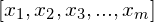where m is the number of predictor variables.

Let y be the response variable. y is a numerical value. We don’t know which value y is taking yet. We are trying to estimate y. Let’s call y’ as our estimation of y.

A linear regression is represented by a list of value, called w.

w =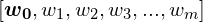.

Hence, our estimation of y, which is y’, is computed using the formula: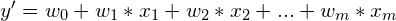.

To make it more simple, we can define an imaginary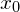which always equals 1. Hence the formula can be re-written as: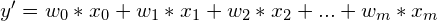.

In Linear algebra point of view, it is just a multiplication of 2 matrices: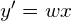And as we want our prediction to be as precise as possible, we would need to find w such that the difference between y and y’ is as small as possible (y = y’ is the best choice), for all samples in our dataset (that is, in the ice-cream example, for all days that we have record of temperature, humidity, and ice-cream sales).

Let’s take a look at my above prediction. Recall that I claimed:

Number of sales tomorrow = 100 + 15 * tomorrow’s temperature – 3 * tomorrow’s humidity.

So, for the first day in the dataset, my estimation of the number of ice-cream sales on that day is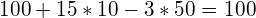. The actual #ice-cream sold that day is 160. So my prediction got error = 60 on the first day.

Continue computing for the next days. I got error 90 on the 2-nd day, 100 on the 3-rd and 105 on the 4th day. So my total error is 60 + 90 + 100 + 105 = 355. Quite bad, right?

Okay, but maybe my guess is still better than my neighbor’s, who knows? Let’s compute the error of his formula! … Yes, his total error is 690, much worse than mine. I’m lucky today.

However, my regression model above gives an error of 355, which is still not good enough. So far, I only guessed the model myself, I didn’t do anything logical to optimize my model, so this model is probably not the best one. In the following blogs, we will say more above how to find the best regression model, logically and rationally. So, stay tuned!

Questions:

1. I understand the above formula is a linear combination, so can you provide some samples of non-linear combination?

2. So linear regression can only work well if the response value is a linear combination of the predictors, right? So we should not use linear regression if the response is not a linear combination of the predictor?

3. According to what you said, I understand that linear regression is the most basic machine learning model, so it would not be used in practice, right? In practice, only the more complicated and advanced algorithms are used, like Deep learning or something?

1. Yes, let me give some examples of non-linear regression:

(1)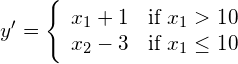(2)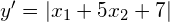.

(3)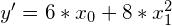.

The above 3 formulas are not linear regression, because they are not in the form of a linear combination, and can not be transformed to the form of a linear combination of the predictors.

By transforming to the form of linear combination of predictors, I mean some thing like this: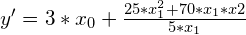which is not currently in the form of linear combination, but can be transformed to: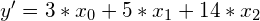.

The transformation above maybe a bit too simple, but you got the point. Any formula that can be equivalently transformed into a linear form can be called a linear regression formula.

A note on the formula (3) above: even though it is not a linear regression, we can easily modify to make it a linear regression by creating a new predictor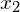, and set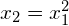. Hence, the formula can be written in the form: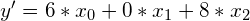,

which is a linear regression formula.

2. Let me answer this question in both theory and practical point of view.

In theory, it is true that: an assumption of Linear regression is that the response variable should be, in fact, a linear combination of predictor variables. If this is not the case, the model is likely to perform badly.

In practice, this is not entirely true. The key lies in the fact that we can do some hacks to the predictor variables. Look at my note on the formula 3 of my answer to the first question above. Originally, the response value has a quadratic relationship with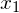(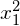). To make the linear regression works, we created a new predictor, with is, and set. This is a method (or, you may call it a cheat) to introduce non-linear relationship to linear regression. Hence, my answer is: in case the relationship between response variable and predictors are non-linear, if you can, by any means, introduce those non-linear relationships to the linear regression, then the linear regression model can still work well.

3. In the last several years, everyone is talking about Deep learning or Deep neural networks. We have to admit that Deep learning have made an extraordinary evolution in the field of Machine learning, and have been creating many breakthroughs.

A small note on this: inside Deep learning (or Deep neural networks), runs many many linear regression formulas. So it’s impossible for us to understand Deep learning without grasping Linear regression first.

Back to the questions, is Linear regression used in practice? My answer is: Yes. Deep learning, even though being very strong on giving precise prediction, still have its own drawbacks. One of those is the need of very large number of samples. Another is, to date, it is still very hard to interpret the results given by Deep nets. We will be talking more about this in the following posts, when I discuss the pros and cons of Linear regression and Deep learning. So, I hope you can bear with me for now. Believe me, Linear regression is very useful, in both theory and practice.

You can find the full series of blogs on Linear regression here.